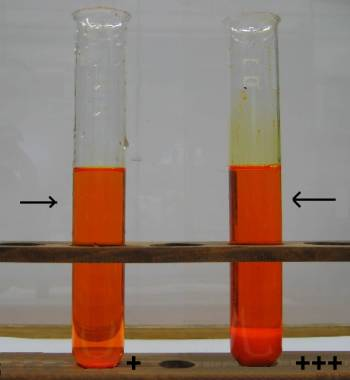Search:

# The heterogeneous equilibrium

## A dissolved solid in contact with the pure solid

### Experiment

Two test tubes contain potassium dichromate, an orange cristalline solid. In the first tube there is a small quantity, in the second more. The same volume of water is added to the two tubes, then the tubes are shaken in order to establish equilibrium. Here are the results:The intensity of coloration in the aqueous phase (therefore the molarity of dichromate) seems to be identical in the two tubes.

### Interpretation

In the aqueous phase, potassium dichromate is dissociated to ions. So we have the equilibrium: $K_2Cr_2O_7(s)$ $\leftrightarrows$ $2K^+(aq)$ $+$ $Cr_2O_7^{2-}(aq)$ As dichromate occurs alone in the lower solid phase, its concentration $c$ $=$ $[K_2Cr_2O_7(s)]$ is constant in that phase. We apply the law of mass action: $\frac{[K^+]_{aq}^2[Cr_2O_7^{2-}]_{aq}}{[K_2Cr_2O_7(s)]}$ $=$ $K$ $\frac{[K^+]_{aq}^2[Cr_2O_7^{2-}]_{aq}}{c}$ $=$ $K$ $[K^+]_{aq}^2[Cr_2O_7^{2-}]_{aq}$ $=$ $K\cdot c$ $[K^+]_{aq}^2[Cr_2O_7^{2-}]_{aq}$ $=$ $K_s$ Which confirms that the intensity of coloration in the aqueous phase (therefore the molarity of dichromate) is constant!

### Generalisation

When a ionic substance $(X^{n+})_m(Y^{m-})_n$ is in equilibrium between the aqueous phase and a solid phase, $(X^{n+})_m(Y^{m-})_n$ $\leftrightarrows$ $m X^{n+}$ $+$ $nY^{m-}$ and when it stays pure in the solid phase, we have: $[X^{n+}]^m[Y^{m-}]^n$ $=$ $K_s$ $K_s$ is a constant at a given temperature and is called solubility product .